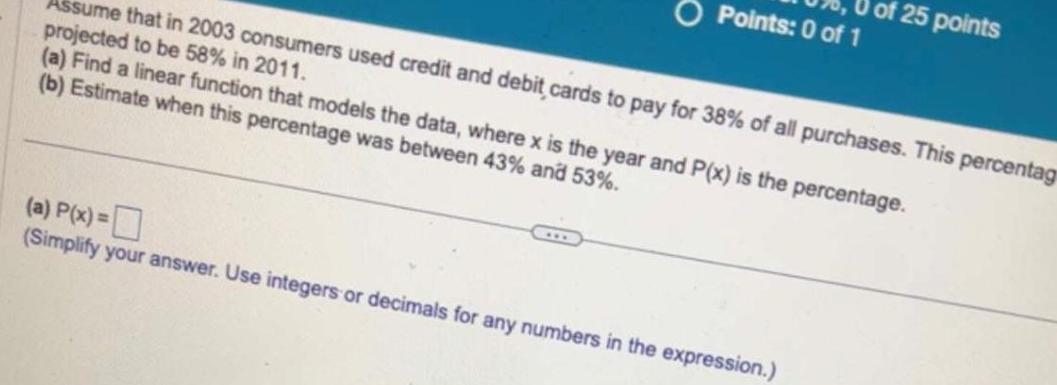Question:

# Assume that in 2003 consumers used credit and debit cards to

Last updated: 9/19/2023Assume that in 2003 consumers used credit and debit cards to pay for 38 of all purchases This percentag projected to be 58 in 2011 a Find a linear function that models the data where x is the year and P x is the percentage b Estimate when this percentage was between 43 and 53 Points 0 of 1 a P x Simplify your answer Use integers or decimals for any numbers in the expression of 25 points